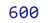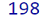# How to find the sum of an array of numbers?

Given an array, we need to find the sum of the numbers in that array.
Submitted by Pratishtha Saxena, on June 18, 2022

There are different ways to sum the numbers in an array. Some of them are discussed below.

1. Using reduce() Method
2. Using Loops

## 1) Using reduce() Method

This method in JavaScript, executes a function provided in it for the array elements. This function is called reducer. This function executes for each element in the array. It returns a single value, in this case will be sum of the numbers of the array.

Syntax:

```array.reduce(function(total, currentValue, currentIndex, arr), initialValue)
```

The terms defined inside the bracket are the parameters that reduce() method accepts. It is not necessary to pass all the parameters every time. But the total and currentValue have to be passes.

Example 1:

```<script>
let numbers = [100,200,300];

result = numbers.reduce((total, current) => total + current, 0);
console.log(result);
</script>
```

Output:## 2) Using Loops

The next way of getting the sum of the numbers in array is the basic method by applying the logic through loop. By using for loop, each element of the array can be iterated. It is then added to the already declared variable. The loop runs throughout the array and sums all the elements in that variable.

Example 2:

```<script>
let numbers = [22, 61, 74, 32, 9]
let sum = 0;

for (let i = 0; i < numbers.length; i++) {
sum += numbers[i]
}

console.log(sum)
</script>
```

Output:Preparation

What's New

Top Interview Coding Problems/Challenges!